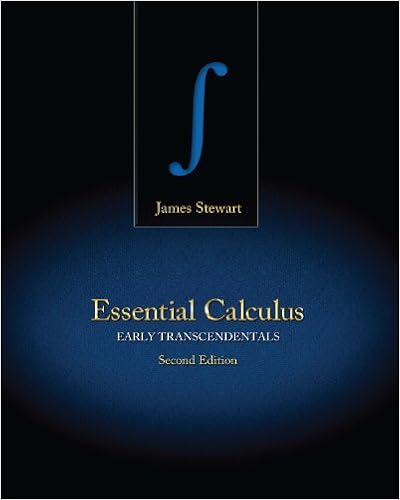Find an equation of the plane passing through the...

• Notes
• 5

This preview shows page 1 - 2 out of 5 pages.

We have textbook solutions for you!
The document you are viewing contains questions related to this textbook.The document you are viewing contains questions related to this textbook.
Chapter 13 / Exercise 1
Essential Calculus: Early Transcendentals
StewartExpert Verified
Math 223, Final Exam Review This review sheet is by no means a complete study guide for the final exam . To review all of the relevant topics, you should also study quizzes, exams, and homework problems. 1. Find an equation of the plane passing through the points P = (1 , - 1 , 2) , Q = (2 , 1 , 3) and R = ( - 1 , 2 - 1) 2. Given the vectors v = h 1 , 1 , 0 i and u = h 2 , 1 , - 2 i , find (a) k v k (b) u × v (c) v × u (d) u · v (e) The angle between v and u 3. Find the first order partial derivatives of the following functions (a) g ( r, θ ) = r 2 cos θ + r sin 2 θ (b) f ( x, y ) = 1 2 ln( x 2 + y 2 ) + tan( y/x ) 4. Find the second order partial derivatives of g ( x, y ) = e x + y sin x 5. Test the function f ( x, y ) = x 3 + y 3 + 3 x 2 - 3 y 2 for local maxima, minima, and saddle points. Find the value of the function at these points. 6. Find the absolute max and min of f ( x, y ) = y 2 - xy - 3 y + 2 x on the region bounded by the lines x = ± 2 and y = ± 2 7. Find the absolute max and min of f ( x, y ) = 2 x 3 + y 4 subject to the constraint x 2 + y 2 = 1.
11. Find the center of mass of a solid of constant mass density that is bounded below by z
We have textbook solutions for you!
The document you are viewing contains questions related to this textbook.The document you are viewing contains questions related to this textbook.
Chapter 13 / Exercise 1
Essential Calculus: Early Transcendentals
StewartExpert Verified
•••